V.6 No 1 77 The problem of physical time in today physics7.1.3. The grounds to transform the time in relativistic conception Proceeding from the necessity to give separately the equation of transform for time intervals, it is important to make sure how much grounded is such transform in the relativistic conception. It will be easy, noting that Einstein tried to do so already in his work of 1905. Let us follow the sequence of Einstein’s derivation, to see how he interrelated the paces of time. “If we put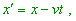(7.14)
 it will be clear that to the point resting in the frame k, a definite, independent on time set of values x', y, z  will belong. We will first determineas a function of x', y, z, t . For it, we have to express with some relationships thatin its meaning is nothing else than an amount of readings of clock resting in the frame k which are synch in accordance with the rule stated in the section 1” [4, p. 14]. Let us make a period in order to understand better, which parameters relate to which frame. Some time before this citation, Einstein described both frames so: “Let in the ‘resting’ space we have two coordinate systems, each with three mutually perpendicular axes that originate from one point… Let now to the coordinate origin of these systems ( k ), the (constant) speed v is given in direction of growth of the axis x of another resting frame ( K ); this speed is passed also to the coordinate axes, as well as to the corresponding scales and clocks. Then to each moment of time t of the resting system ( K ), a definite location of the axes of the moving system corresponds… To each set of values x, y, z, t  that fully determine the place and time of the event in the resting frame, the set of values,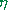,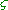,,corresponds and establishes this event in the system k” [4, p. 13]. According to this citation, the set of parameters x, y, z, t  corresponds to the resting frame K and the set of parameters,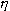,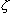,corresponds to the resting frame k. Then the question arises: to which frame x'   relates in (7.14)? The expression (7.14) has an appearance of Galilee transform, which predicts no transforms of spatial parameters. The same we read in the Einstein’s phrase “ that to the point resting in the frame k, a definite, independent on time set of values x', y, z will belong”. Thus, to be not confusing the parameters of frames, it would be more logical to write (7.14) as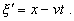(7.15)
 However, with it other questions arise. First, if Einstein wrote (7.14), meaning (7.15), he would have to account the transform coefficient of the frame k and to write so as he wrote it further: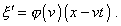(7.16)
 As he did not so, he factually says us in this consideration that there are no transforms of spatial parameters along the motion. And if further, basing on the postulate of lightspeed constancy, he comes to the opposite, in this way he again contradicts the statement of problem. Second, it immediately follows from (7.15) that the timein the frame k depends not only on the parameters of K, as it is usual in the transform equations, but on three parameters of K and one parameter of k, namely – on(', y, z, t ) . In other words, yet having not proven the truth of transforms, Einstein already introduces dependence of time in the moving frame on the spatial coordinates of same frame; this violates the basic property of time and the condition of fully synch time in the moving frame introduced just by himself. It could not make no effect on his following considerations. “Let from the coordinate origin of the system k (i.e., from the point= 0  – Authors) at the moment of time0  the beam of light is sent along the axis X to the point  x' (i.e., to the point'  resting relative to k – Authors) and is reflected from it at the moment of time1  back, to the coordinate origin, and arrives there at the moment of time2 ; then there has to exist the relationship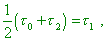(7.17)
 (which evidences of isotropy of lightspeed in k – Authors) or, writing out the arguments of functionand applying the principle of constant speed of light in the resting frame, yield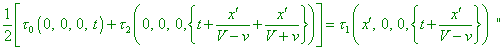(7.18)
 [4, p.14]. Here we have to stop again to analyse (7.18). First, we see from it that all values ofin its left and right parts depend in the Einsteinian formula on some indefinite parameter t, while the values0,1,2  determine the specific moments of radiation, reflection and receipt of beam in k. It seems to be insignificant and we may put, instead an indefinite t, a specific value t0   which has to be there by its meaning, as quite specific moment t0   in the frame K naturally corresponds to the moment of radiation0   in k. And if it is insignificant for relativists, let us do so. With it (7.18) will take the form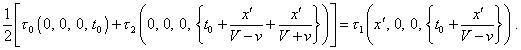(7.19)
 Second, in the second summand of left part and in the right part we see that to the initial moment t0   of the frame K there are added the terms containing x'  – the parameter which, as we saw above, relates to another frame k. Is it occasional? Further we will see – no. But anyway the time t0   from the radiation to the moment of reflection t0 , just as the time from the moment of reflection t1   to the moment of reception, cannot be in K determined by x'  in the appearance in which it is introduced in the above relationships, and the more to be added to the moment t0 . So we have to correct also (7.18) and to put into it a particular value of x1  which we cannot find more accurately in Relativity because of indefinite degree of time transform ( at this level of Einstein’s proof of his hypothesis). While in classical formalism, just as with (7.14), we can write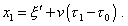(7.20)
 The only thing which we could refine in (7.20), given (7.16), is to add an indefinite transform coefficient for spatial parameters and to account that according to (7.17), the light is propagated isotropically in the frame k, and the value'    is known by the statement of problem: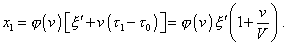(7.21)
 With these refinements, the appearance of summands in the above terms with time of (7.18) will naturally change. To see this change, we have to account that the path from radiator to the mirror, from the point of K (due to motion of the reflection point'  relative to this frame) will be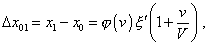(7.22)
 and the path from the mirror to the receiver from the point of frame K is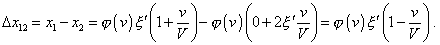(7.23)
 Given (7.22) and (7.23) and the postulate of lightspeed constancy in all frames declared by Einstein, (7.19) will take an appearance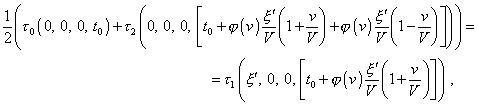(7.24)
 or otherwise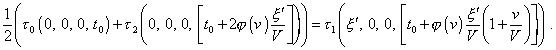(7.25)
 In this expression we immediately see that'   and t0    are some constant parameters, and the presence of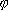(v)  makes even more difficult to find(t) . From this point, it is interesting, of course, how the author will finish his long way to (7.18), or rather to (7.25) which would stand instead it, should we keep the mathematical operations correct in modelling of physical processes. “If we take x' infinitesimal, it follows from this (from (7.18) – Authors) that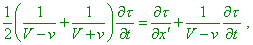(7.26)
 or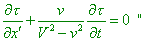(7.27)
 [4, p. 14]. We see from (7.26) and (7.27) that all ‘slips of the pen’ that we corrected in the course of Einstein’s derivation appeared in fact quite realised substitutions. We see in his formulas how the derivatives in time t appeared which, as we saw above, is not a variable but only determines the moment of beam radiation in K. Now the derivative in x'  appeared also, though this parameter was constant, too, and it did not become variable because it was taken small, while Einstein will integrate over it. This parameter only determines the location of reflection point in the frame k, nothing else. Thus, we can ascertain that Einstein’s attempt to substantiate the necessity of time contraction through comparing the time intervals in mutually moving frames reduced to juggling with formulas and symbols. In reality, the very fact of time contraction, and of anisotropic time transform that follows from Lorentz transforms, is absurd. Even if we consider time as relative time that means the correlation of sequence of events with some specific periodic process, it is enough to take as an example a simple pendulum to make sure how senseless are such transformations. Actually, let us take a plane pendulum and try to check the processes in the moving frame. According to the relativistic formalism, dependently on the orientation of plane of swing relative to the frame’s motion, its mass, elasticity of springs and radius of swing have to vary, and the variations have to be detectable straight in the moving frame. At the same time, we can detect the events at some chosen point equally at most different orientations of periodic process, as for us the matter of importance is, at a point where we observe the event, the body – carrier of periodic process to coincide with the studied body (to meet it). Around this point the plane of pendulum can take an arbitrary position in space. And always the pendulum will come to the point of meeting with the studied body. And, as we always can juxtapose to any specific point where the event occurred many differently oriented pendulums, the choice of true coefficient of time transform becomes indefinite and, hence, insolvable. This is why Einstein’s derivation basically could not give a result. And Einstein understood, he cannot prove the time transformation, basing on particular model of intervals transmission. So in other his work, “The principle of relativity and its corollaries in today physics” , he gives another proof of interrelation of times. Here he already does not try to direct a beam but simply writes: “We have put forward the task to find the relationships that interrelate these two sets of coordinates. Using the homogeneity of time (?! – Authors) and space, we can show that these relationships have to be linear, i.e. t and t'  are interrelated as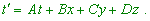(7.28)
 …, coordinates x, y, z, x', y', z'   are interrelated as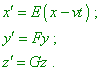(7.29)
 To find the constants A, B, C, D, E, F, G  in (7.28) and (7.29), note that according to the principle of lightspeed constancy, the speed of propagation has one and the same value c relative to both frames, i.e., equations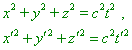(7.30)
 are equivalent. Substituting in the second equation t', x', y', z'   by their values from (7.28) and (7.29) and comparing with the first equation, yield the transform formulas…” [17, p. 152–153]. We see from this citation that Einstein simply substituted the derivation of transform formula for temps of time by the proof that the 4-interval based on postulate, not on the experimentally checked fact, is invariant for the Lorentz transforms. But when comparing the pace of time, we factually compare the times at different points of inclined plane of events; this discrepancy remains and, as we already said, it cannot be solved by the methods with which relativists try to solve it.

Contents: / 53 / 54 / 55 / 56 / 57 / 58 / 59 / 60 / 61 / 62 / 63 / 64 / 65 / 66 / 67 / 68 / 69 / 70 / 71 / 72 / 73 / 74 / 75 / 76 / 77 / 78 / 79 / 80 / 81 / 82 / 83 /